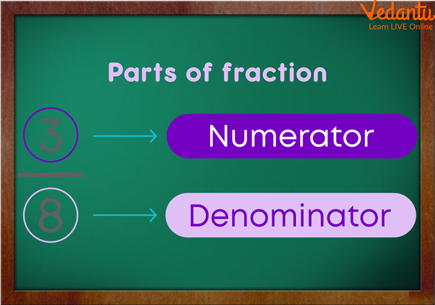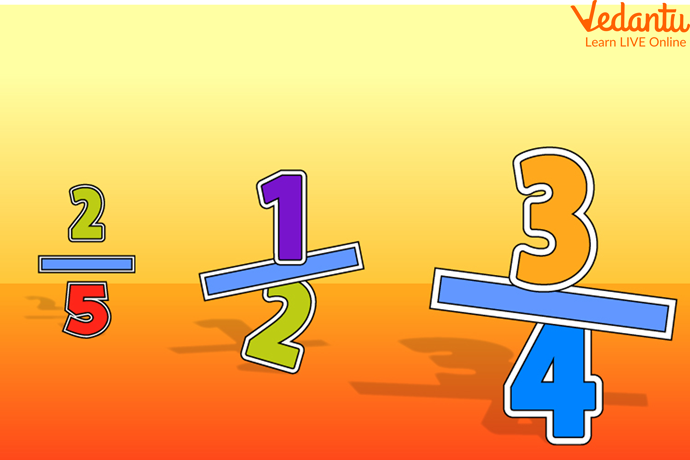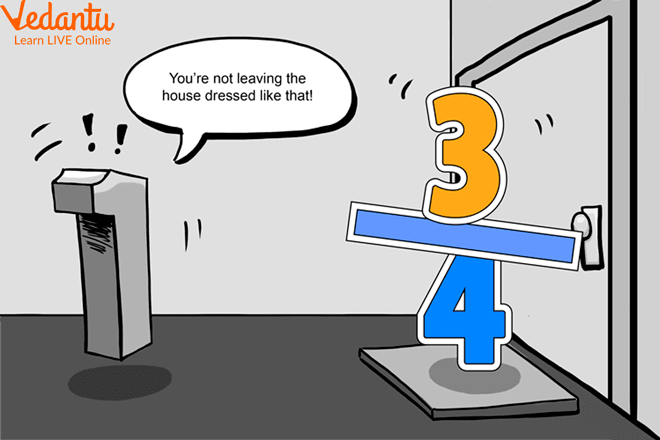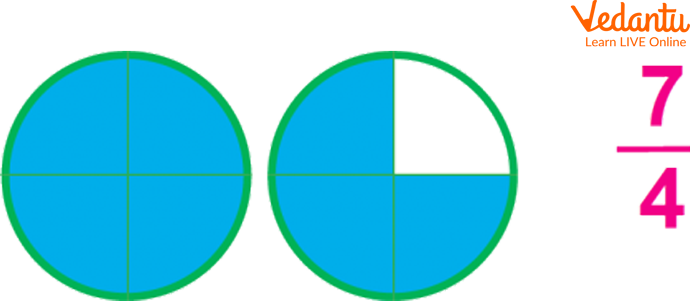Courses
Courses for Kids
Free study material
Offline Centres
More

# Vulgar Fraction with Solved ExamplesLast updated date: 11th Dec 2023
Total views: 147k
Views today: 2.47k## What is a Vulgar Fraction?

Do you know another name for a common fraction? A fraction in which the numerator and denominator are both integers. Vulgar fractions are simply two integers placed above and below a fraction bar (the numerator and denominator). A vulgar fraction, also known as a common fraction or fraction, is a fraction written in the conventional form, with one integer number written above the next and a line between them.The Numerator and The Denominator

## What are Numerators and Denominators?

The numerator predicts the total number of selected equal fractions. Whereas the denominator demonstrates the whole number of equal parts. A circular wheel is distributed into six equal sections. This situation can be represented as a $\dfrac{5}{6}$ fraction, the numerator 5 represents the number of the equivalent shaded portion taken out from the set of 6 equal amounts, and the denominator 6 means that 6 equal parts make up a whole unit.

## Vulgar Fractions FactsExamples of The Vulgar Fraction

• If the numerator and denominator are multiplied or divided by the same number, the value of a vulgar fraction remains the same.

• A fraction can be lowered only when the numerator and denominator share the exact divisors.

• The fractions can be added by adding their numerators if their denominators are the same; to subtract them, take the numerator of the subtrahend and divide it by the numerator of the minuend; to multiply two fractions by fractions, multiply the numerators for the product's numerator and the denominators for the product's denominator.Fraction

• Finally, multiply the integer by the reciprocal of the particle to divide it by the fraction. The denominator cannot contain 0; it should be noted. Since denominators are also divisors, no integer may be split by 0, and the same rule applies to irrational numbers. Therefore, the denominator must be rationalised.

## What is an Improper Fraction?Improper Fraction

When the numerator is less than the denominator, the fraction is less than one and is referred to as a proper fraction. For example, $\dfrac{1}{2}$ and $\dfrac{5}{11}$ are correct fractions. If the numerator equals the denominator, the fraction is equal to unity, such as $\dfrac{7}{7}$. If the $\dfrac{200}{100}$ numerator is greater than the denominator, the fraction is more significant $\overline{100}$. The fraction is known as an improper fraction.

## Converting Decimal to Vulgar Fraction

In the denominator, put 1 under the decimal point and annex with it as many zeros as is the number of digits after the decimal point. Then, remove the decimal point and reduce the fraction to the lowest terms.

## Solved Examples on Vulgar Fraction

Q1. Express 0.18 as a Vulgar Fraction.

Ans: x = 0.18

Multiply both aspects by 10 (Since the number of digits without a bar is 1)

10x = 1.8

$10 x=1.88 .... . .(\text { i) }$

$10 \times 10 x=1.88 \ldots . . \times 10$ (Since number of digits having bars is 1

$100 x=18.8 \ldots . . \ldots \text { (ii) }$

Subtracting (i) from (ii)

$100 x-10 x=18.8-1.8$

$90 x=17$

$x=\dfrac{17}{90}$

Therefore, the vulgar fraction $=\dfrac{17}{90}$

## Summary

A vulgar fraction, also known as a common fraction or fraction, is a fraction written in the conventional form, with one integer number written above the next and a line between them. Examples of vulgar fractions include 1/2, 5/11, and 200/100. A vulgar fraction is a part of unity or several equal parts of unity. A vulgar fraction is one that is based on ordinary or everyday arithmetic, as opposed to these highfalutin decimal things, which were originally called decimal fractions. It specifically refers to one in which two whole numbers (the numerator and denominator) are placed above and below a horizontal line. Neither part can be zero.

## FAQs on Vulgar Fraction with Solved Examples

1. What is a vulgar fraction?

A vulgar fraction, also known as a common fraction or fraction, is a fraction written in the traditional manner, with one number (integer) above another (integer) separated by a line. $\dfrac{1}{2}, \dfrac{5}{11}$, and $\dfrac{200}{100}$ are examples of vulgar fractions. A vulgar fraction is a part of unity or several equal parts of unity in mathematics. The denominator of the fraction is the number that indicates how many parts a unit is divided into; the numerator of the fraction is the number that indicates how many parts are taken.

2. What do you understand by the terms numerator and denominator?

Numerators and denominators are essential values that help understand the nature of a fraction. Understanding these values helps better understand the given fraction and see whether it is a proper or an improper fraction. The numerator and denominator are fractional parts separated by a horizontal bar called a vinculum. The numerator is the fraction's upper part, and the denominator is the fraction's lower part. When we divide the numerator and denominator, we treat the numerator as a dividend and the denominator as the divisor.